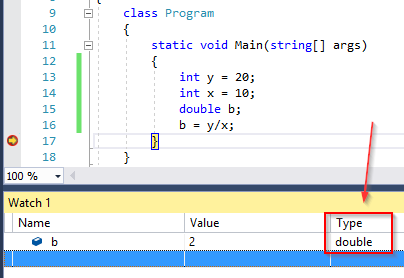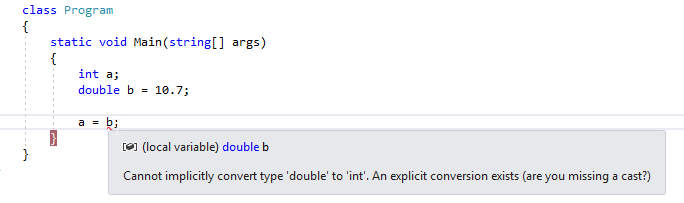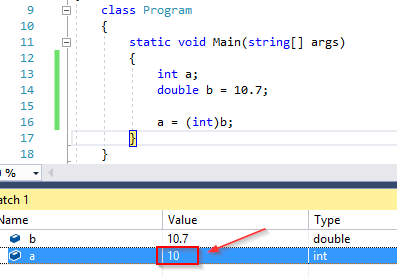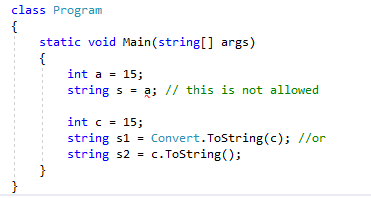In C#, data can be converted from one type to another by using an implicit conversion (automatic) or an explicit conversion (we can choose how it’s done).

For the complete navigation of this series check out: C# Back to Basics.

So, let’s talk more about that.

## Implicit Conversion

Many different data could be interpreted by using different types. For example, number 74 can be interpreted as an integer but also as double (74.0). There are two situations in which implicit conversion applies.

The first one is when we calculate an expression. The compiler automatically adapts data types that we use in that expression:

```class Program
{
static void Main(string[] args)
{
double b = 12.45;
int x = 10;
b = b + x;
}
}
```

In the code above the `b` variable is of type double and `x` is of type int. In the expression `b + x`, the compiler implicitly converts `x` from int to double and then it assigns a result to the `b`.

The second situation for a conversion is when the compiler stores the result to a variable:In this example, we see that both `x` and `y` are of the type int, but the result is of the type double.

Now, let’s pay attention to this example:

```int x = 21;
int y = 5

double b = x/y;```

What do you think, which value is stored in the variable `b`?

The answer: “The result is 4.2” is not correct. Of course, now goes the question: “But why”?

The compiler calculates the right side expression first and only then converts that result into double. So, the right side expression `x/y`consist of integer numbers, thus the result is an integer number as well, in this example, it is 4 (the value is truncated). After that calculation, compiler converts result into a double and assigns the value to the b variable:We can fix this if we want, by using the explicit conversion on either `x` or `y` variable in the expression.

## Explicit Conversion

For the explicit conversion, we need to write additional code to convert one type to another. We have two different ways, by using a cast operator or by using the `Convert` class.

Let’s look at the following example:The compiler complains about an invalid conversion. What we are missing here is the cast operator, so let’s use it:By using the cast `(int)`conversion, we can safely cast our data types and the compiler approves of that. But what we can see is that our result is not what we would expect it to be. But this is the correct result. It is very important to understand that the cast operator can shrink data when we convert the type with the larger value scope to a type with the smaller value scope. Like we did with the double to int conversion for example.

Now we can apply the cast operator on our example from the Implicit Conversion part, to get the right result:

```class Program
{
static void Main(string[] args)
{
int x = 21;
int y = 5;

double b = (double)x / y;
}
}```

The result is going to be 4.2.

## Using the Convert Class

As we said, we can use the Convert class with its static methods, to explicitly convert one base type to another base type:## Conclusion

Excellent.

We have learned about type conversion and how implicit and explicit conversion behaves in C#.

In a next post, we are going to talk about Linear Structures in C#.